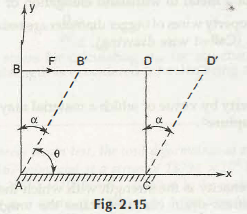Shear stress takes place due to shear strain.

At this time,

Shear stress/ shear strain = G,

Here, G is called the shear modulus or modulus of rigidity.

txy / ᵞxy = G

txy  = G . ᵞxy

Now, in this following figure there is a rectangle box ABCD. After applying the force on AC the new position after distorting an angle is AB’CD’.ᵞxy = DD / CD = tan a

= a (value of a is very small)

You can easily notice here that the angle θ is the new angle after acquiring the position of AB’CD’.  The remaining value of the original angle is denoted here with α.

Now, by applying the definition of strain, it can be defined as

ᵞxy = 900 – θ = a ,

No matter how small the angle is, but it is very important to know that the base AC remains same and the formation of new sides is AB’, B’C’ and CD’.

Links of Next Mechanical Engineering Topics:-### Customer Reviews

My Homework Help
Rated 5.0 out of 5 based on 510 customer reviews at